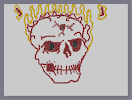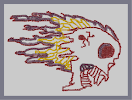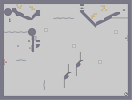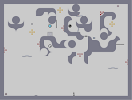### Flaming Skull v.2 HOT HEADHover over the thumbnail for a full-size version.

Author metallica_rulz author:metallica_rulz hard rated 2009-04-15 2009-04-15 4 by 5 people. \$Flaming Skull v.2 HOT HEAD#metallica_rulz#none#00000000000000000000000000000000000000000000000000000000000000000000000000000000000000000000000000000000000000000000000000000000000000000000000000000000000000000000000000000000000000000000000000000000000000000000000000000000000000000000000000000000000000000000000000000000000000000000000000000000000000000000000000000000000000000000000000000000000000000000000000000000000000000000000000000000000000000000000000000000000000000000000000000000000000000000000000000000000000000000000000000000000000000000000000000000000000000000000000000000000000000000000000000000000000000000000000000000000000000000000000000000000000000000000000000000000000000000000000000000000000000000000000000000000000000000000000000000000000000|5^309,290!12^293,457!12^299,462!12^307,468!12^316,476!12^322,490!12^330,498!12^346,498!12^364,498!12^375,498!12^392,498!12^402,497!12^414,493!12^424,482!12^437,487!12^447,492!12^476,464!12^483,444!12^480,432!12^475,420!12^517,279!12^511,270!12^527,297!12^521,287!12^510,258!12^517,249!12^512,237!12^502,228!12^498,226!12^494,221!12^489,216!12^488,213!12^486,205!12^496,215!12^497,211!12^500,202!12^502,189!12^502,174!12^502,170!12^500,164!12^497,153!12^493,146!12^486,137!12^480,131!12^474,126!12^470,123!12^463,121!12^457,120!12^450,118!12^445,114!12^215,144!12^191,177!12^190,179!12^188,185!12^186,195!12^185,204!12^185,214!12^185,227!12^187,246!12^189,260!12^194,270!12^201,277!12^211,287!12^217,290!12^229,298!12^234,270!12^237,275!12^237,284!12^236,286!12^236,295!12^234,298!12^229,306!12^224,311!12^216,321!12^212,330!12^212,335!12^212,344!12^212,347!12^212,352!12^220,355!12^227,355!12^231,355!12^231,358!12^233,363!12^233,366!12^233,372!12^234,378!12^234,382!12^238,384!12^241,385!12^246,387!12^251,387!12^261,387!12^267,387!12^276,387!12^281,387!12^288,385!12^291,384!12^297,383!12^303,382!12^306,381!12^312,381!12^318,386!12^320,390!12^321,393!12^321,396!12^316,397!12^282,392!12^282,393!12^282,403!12^282,419!12^285,424!12^294,425!12^296,425!12^306,426!12^311,425!12^317,415!12^318,408!12^318,394!12^312,427!12^313,428!12^294,456!12^292,453!12^285,448!12^279,444!12^273,437!12^267,430!12^265,426!12^261,421!12^260,417!12^258,412!12^256,405!12^250,400!12^247,395!12^247,392!12^425,328!12^425,324!12^425,318!12^425,315!12^422,311!12^422,310!12^418,305!12^415,298!12^411,292!12^411,287!12^411,281!12^405,272!12^402,268!12^400,264!12^393,257!12^393,257!12^394,257!12^394,265!12^394,267!12^394,275!12^394,280!12^394,286!12^392,290!12^389,294!12^387,298!12^386,299!12^383,306!12^382,314!12^384,320!12^389,322!12^395,324!12^397,329!12^398,333!12^398,338!12^400,340!12^408,338!12^406,334!12^419,331!12^422,332!12^404,344!12^402,333!12^402,327!12^402,321!12^398,319!12^396,319!12^392,318!12^390,318!12^384,312!12^384,306!12^390,300!12^390,300!12^390,294!12^390,288!12^402,276!12^402,276!12^402,276!12^402,288!12^402,294!12^402,306!12^402,306!12^402,300!12^402,294!12^402,288!12^402,276!12^396,306!12^396,306!12^396,300!12^396,294!12^396,288!12^390,312!12^390,306!12^384,312!12^384,318!12^396,324!12^402,336!12^420,324!12^420,318!12^420,312!12^414,312!12^414,306!12^408,294!12^408,294!12^402,288!12^402,282!12^396,270!12^396,264!12^396,258!12^408,306!12^408,300!12^408,300!12^408,294!12^408,288!12^408,288!12^408,282!12^408,282!12^396,282!12^396,276!12^396,276!12^396,270!12^402,276!12^402,276!12^402,270!12^408,276!12^414,318!12^414,318!12^414,306!12^414,300!12^408,294!12^408,306!12^408,300!12^408,300!12^408,300!12^414,300!12^408,294!12^414,294!12^402,300!12^420,324!12^409,311!12^395,311!12^410,245!12^414,245!12^417,245!12^420,251!12^422,253!12^423,257!12^424,264!12^424,270!12^429,275!12^434,278!12^437,281!12^439,285!12^445,292!12^452,294!12^459,294!12^464,294!12^467,292!12^472,291!12^472,291!12^475,290!12^479,286!12^480,281!12^482,277!12^483,271!12^485,260!12^487,253!12^487,248!12^487,246!12^487,242!12^487,236!12^487,234!12^482,232!12^477,231!12^470,231!12^466,231!12^459,235!12^453,235!12^448,236!12^441,237!12^433,238!12^424,243!12^421,243!12^416,244!12^410,244!12^485,234!12^490,234!12^493,237!12^495,240!12^497,242!12^502,246!12^502,250!12^502,253!12^480,288!12^478,291!12^475,296!12^472,299!12^467,303!12^465,306!12^434,286!12^436,288!12^438,291!12^438,296!12^439,298!12^440,304!12^444,305!12^446,306!12^380,242!12^378,243!12^372,248!12^367,255!12^366,256!12^362,264!12^362,266!12^358,273!12^356,279!12^355,284!12^355,291!12^355,298!12^355,303!12^355,307!12^350,315!12^346,318!12^345,319!12^350,301!12^340,305!12^335,307!12^326,308!12^322,308!12^311,308!12^303,308!12^301,308!12^295,305!12^291,304!12^288,303!12^285,300!12^281,299!12^278,295!12^273,289!12^270,283!12^269,280!12^269,278!12^269,274!12^269,268!12^271,260!12^275,251!12^275,250!12^282,249!12^291,249!12^297,249!12^306,249!12^314,256!12^317,256!12^327,258!12^336,258!12^349,258!12^360,257!12^360,256!12^345,256!12^270,254!12^260,267!12^251,277!12^260,268!12^266,257!12^258,271!12^253,278!12^249,280!12^266,295!12^266,293!12^266,291!12^269,285!12^289,319!12^286,316!12^283,312!12^279,309!12^278,307!12^276,305!12^275,303!12^261,317!12^261,310!12^263,307!12^265,299!12^251,238!12^251,238!12^251,234!12^250,229!12^250,223!12^250,219!12^249,226!12^255,226!12^263,224!12^272,222!12^284,217!12^292,217!12^302,217!12^312,217!12^321,217!12^329,217!12^448,209!12^449,207!12^456,201!12^464,196!12^467,194!12^479,193!12^530,296!12^523,305!12^529,295!12^525,286!12^476,412!12^476,410!12^476,408!12^475,405!12^475,401!12^476,394!12^479,387!12^481,378!12^488,371!12^492,363!12^494,357!12^500,350!12^503,343!12^506,337!12^510,329!12^514,320!12^512,317!12^288,390!12^288,402!12^288,402!12^288,408!12^288,408!12^288,414!12^288,420!12^288,420!12^294,396!12^288,396!12^282,396!12^282,402!12^282,402!12^282,414!12^282,414!12^282,420!12^282,420!12^282,414!12^294,420!12^294,420!12^294,414!12^294,414!12^294,408!12^294,408!12^294,402!12^294,402!12^294,396!12^294,390!12^294,390!12^294,384!12^300,384!12^300,384!12^300,390!12^300,390!12^300,396!12^300,402!12^300,408!12^300,408!12^300,414!12^300,414!12^300,420!12^300,420!12^300,426!12^306,426!12^306,414!12^306,414!12^306,408!12^306,408!12^306,396!12^306,396!12^306,390!12^312,384!12^312,384!12^306,390!12^306,390!12^306,396!12^306,396!12^306,402!12^306,408!12^306,414!12^312,420!12^312,426!12^312,426!12^312,420!12^312,414!12^312,414!12^312,408!12^312,408!12^312,402!12^312,396!12^312,396!12^312,396!12^312,390!12^306,384!12^306,384!12^306,420!12^288,426!12^282,408!12^318,402!12^320,422!12^322,403!12^318,430!12^318,428!12^319,425!12^318,423!12^320,420!12^320,418!12^320,416!12^320,413!12^321,411!12^321,409!12^480,381!12^478,382!12^474,386!12^467,388!12^462,394!12^462,394!12^463,402!12^464,409!12^467,414!12^470,421!12^470,421!12^469,417!12^469,411!12^469,407!12^469,401!12^469,400!12^469,396!12^472,393!12^473,391!12^464,392!12^464,397!12^466,406!12^472,415!12^473,421!12^477,422!12^478,418!12^478,412!12^478,405!12^481,398!12^482,393!12^482,385!12^487,378!12^489,372!12^473,426!12^473,426!12^475,427!12^475,429!12^476,429!12^475,428!12^473,419!12^473,408!12^468,400!12^465,395!12^473,391!12^481,387!12^472,393!12^464,393!12^336,449!12^333,447!12^330,442!12^326,437!12^325,432!12^323,426!12^325,418!12^327,418!12^333,418!12^340,418!12^349,416!12^349,416!12^351,416!12^357,416!12^360,416!12^364,418!12^377,421!12^373,420!12^376,420!12^380,420!12^386,420!12^392,420!12^393,420!12^405,420!12^409,420!12^411,419!12^418,419!12^421,419!12^421,419!12^434,417!12^438,416!12^444,412!12^446,411!12^345,419!12^345,419!12^345,423!12^346,430!12^346,435!12^349,442!12^367,446!12^367,445!12^367,442!12^367,439!12^367,434!12^367,430!12^367,426!12^399,426!12^399,425!12^398,423!12^397,425!12^397,429!12^397,431!12^397,435!12^397,439!12^397,443!12^424,420!12^424,420!12^424,423!12^425,430!12^427,436!12^427,442!12^438,415!12^440,417!12^443,423!12^443,426!12^444,432!12^445,411!12^448,412!12^450,418!12^451,421!12^448,409!12^452,408!12^453,409!12^455,415!12^460,419!12^328,415!12^328,410!12^329,406!12^330,402!12^332,399!12^335,394!12^345,411!12^345,408!12^347,405!12^348,402!12^349,399!12^349,397!12^350,394!12^366,417!12^367,411!12^367,408!12^367,405!12^367,402!12^367,400!12^368,395!12^400,418!12^399,411!12^399,407!12^399,402!12^399,400!12^399,397!12^421,417!12^420,413!12^418,410!12^417,407!12^417,407!12^417,402!12^415,398!12^435,410!12^435,409!12^432,404!12^432,402!12^429,398!12^428,394!12^428,418!12^446,406!12^445,406!12^445,403!12^444,401!12^444,399!12^442,394!12^441,390!12^452,406!12^458,405!12^459,404!12^453,403!12^453,397!12^452,394!12^452,390!12^264,428!12^267,430!12^271,436!12^280,442!12^280,446!12^285,448!12^288,450!12^293,452!12^294,455!12^298,459!12^300,460!12^307,473!12^314,480!12^315,482!12^317,484!12^323,486!12^324,489!12^324,494!12^334,497!12^338,497!12^344,497!12^351,497!12^355,497!12^360,497!12^365,497!12^369,497!12^376,497!12^381,497!12^389,497!12^394,497!12^399,497!12^406,496!12^410,494!12^414,493!12^416,492!12^418,491!12^419,489!12^421,487!12^424,485!12^435,484!12^437,485!12^439,485!12^441,487!12^445,487!12^463,486!12^463,483!12^469,476!12^469,476!12^470,475!12^473,468!12^474,466!12^476,465!12^477,459!12^477,458!12^478,454!12^480,445!12^480,445!12^480,440!12^480,435!12^428,483!12^431,483!12^438,484!12^441,489!12^445,488!12^453,488!12^463,486!12^467,483!12^481,449!12^488,364!12^501,344!12^502,340!12^502,337!12^506,335!12^509,331!12^515,316!12^521,311!12^521,311!12^520,312!12^513,322!12^511,323!12^505,335!12^499,339!12^494,350!12^490,353!12^489,356!12^526,296!12^526,295!12^526,295!12^525,293!12^522,287!12^522,287!12^517,281!12^515,279!12^512,276!12^509,271!12^508,268!12^507,266!12^506,262!12^506,257!12^510,254!12^514,252!12^514,247!12^514,243!12^512,241!12^507,234!12^504,231!12^500,228!12^496,225!12^496,222!12^496,218!12^496,211!12^496,205!12^496,201!12^498,197!12^498,191!12^500,184!12^486,216!12^486,211!12^486,210!12^484,204!12^482,201!12^478,195!12^472,194!12^469,194!12^466,195!12^460,198!12^453,199!12^448,206!12^502,182!12^501,176!12^501,170!12^501,164!12^499,156!12^498,153!12^494,148!12^487,141!12^480,135!12^471,126!12^352,300!12^350,300!12^344,300!12^335,304!12^330,305!12^324,308!12^319,308!12^313,308!12^272,254!12^276,250!12^281,248!12^291,247!12^296,247!12^303,247!12^309,252!12^314,254!12^318,254!12^329,255!12^337,255!12^344,255!12^350,255!12^354,255!12^342,258!12^332,258!12^325,217!12^320,217!12^314,217!12^307,217!12^301,217!12^297,217!12^292,217!12^287,217!12^271,220!12^265,220!12^258,225!12^256,227!12^414,242!12^420,242!12^428,242!12^434,241!12^439,240!12^446,238!12^454,235!12^463,232!12^451,292!12^460,291!12^258,313!12^264,308!12^264,304!12^269,299!12^251,284!12^255,280!12^256,276!12^259,273!12^260,269!12^262,264!12^264,263!12^234,271!12^238,277!12^238,281!12^238,288!12^238,291!12^232,299!12^227,302!12^223,306!12^221,311!12^220,314!12^218,318!12^218,324!12^218,325!12^213,332!12^213,336!12^213,342!12^213,349!12^214,354!12^270,386!12^270,386!12^265,386!12^262,384!12^255,383!12^247,384!12^315,481!12^313,476!12^309,472!12^305,470!12^298,462!12^227,292!12^221,292!12^219,291!12^216,288!12^211,283!12^209,281!12^202,275!12^202,274!12^197,269!12^193,265!12^190,263!12^187,256!12^186,250!12^186,247!12^184,241!12^183,239!12^183,236!12^183,230!12^183,227!12^183,224!12^183,222!12^183,215!12^183,208!12^183,206!12^184,198!12^184,193!12^185,190!12^189,181!12^189,178!12^190,174!12^214,146!12^218,144!12^484,266!12^324,256!12^324,256!12^267,400!12^299,440!12^328,372!12^298,366!12^281,378!12^287,372!12^468,378!12^477,369!12^460,431!12^471,443!12^463,439!12^474,282!12^474,282!12^486,264!12^480,258!12^480,258!12^480,252!12^480,234!12^480,234!12^480,234!12^474,240!12^480,246!12^480,246!12^480,264!12^480,270!12^474,276!12^468,282!12^462,288!12^456,288!12^450,288!12^444,282!12^444,282!12^438,276!12^432,270!12^426,264!12^426,258!12^426,252!12^426,246!12^426,246!12^438,246!12^450,246!12^456,240!12^468,240!12^474,246!12^480,246!12^480,240!12^474,234!12^456,234!12^450,240!12^444,246!12^438,246!12^450,246!12^462,246!12^462,246!12^462,240!12^462,240!12^462,246!12^474,246!12^474,246!12^474,252!12^474,258!12^474,264!12^474,270!12^468,276!12^468,282!12^468,282!12^468,270!12^468,264!12^468,264!12^468,258!12^468,252!12^468,246!12^462,246!12^462,246!12^462,258!12^462,258!12^462,264!12^462,270!12^462,282!12^456,282!12^456,282!12^456,276!12^468,276!12^468,282!12^468,288!12^474,276!12^480,276!12^474,276!12^462,282!12^462,264!12^456,276!12^462,276!12^474,288!12^468,234!12^474,234!12^462,240!12^468,234!12^456,252!12^456,246!12^456,252!12^456,258!12^456,258!12^456,270!12^456,276!12^456,270!12^456,270!12^456,264!12^456,264!12^462,258!12^462,252!12^462,240!12^450,240!12^444,240!12^444,240!12^462,234!12^486,258!12^450,276!12^444,282!12^444,282!12^450,282!12^450,276!12^450,276!12^444,270!12^444,270!12^444,264!12^444,264!12^444,258!12^444,258!12^438,252!12^438,252!12^432,246!12^426,246!12^426,252!12^444,252!12^444,252!12^444,252!12^450,264!12^444,264!12^450,258!12^450,258!12^450,252!12^438,258!12^426,258!12^426,258!12^432,264!12^432,270!12^444,276!12^444,282!12^444,270!12^438,270!12^438,270!12^438,270!12^432,264!12^432,258!12^432,258!12^432,252!12^432,252!12^456,270!12^450,270!12^444,288!12^450,288!12^438,276!12^444,264!12^432,264!12^414,246!12^426,252!12^432,264!12^432,270!12^438,264!12^444,264!12^438,264!12^426,270!12^450,282!12^420,252!12^486,246!12^456,294!12^432,270!12^420,246!12^360,264!12^360,264!12^354,264!12^348,264!12^342,264!12^336,264!12^336,264!12^336,264!12^330,264!12^324,264!12^318,264!12^318,264!12^312,264!12^312,264!12^306,264!12^300,264!12^300,264!12^294,264!12^294,264!12^288,264!12^288,264!12^282,264!12^282,264!12^276,264!12^276,264!12^276,258!12^276,258!12^282,258!12^282,258!12^288,258!12^288,258!12^294,258!12^300,258!12^300,258!12^300,258!12^306,252!12^306,252!12^300,252!12^294,252!12^294,252!12^288,252!12^288,252!12^282,252!12^282,252!12^276,264!12^276,270!12^282,270!12^282,270!12^288,270!12^294,270!12^294,270!12^300,270!12^306,270!12^306,270!12^312,270!12^312,270!12^318,270!12^318,270!12^324,270!12^324,270!12^330,264!12^330,264!12^336,264!12^354,264!12^354,270!12^348,270!12^348,270!12^342,270!12^336,270!12^330,270!12^306,258!12^306,258!12^324,258!12^336,258!12^354,258!12^354,264!12^318,258!12^312,258!12^312,258!12^348,276!12^348,276!12^342,276!12^342,276!12^342,276!12^336,276!12^336,276!12^330,276!12^330,276!12^324,276!12^324,276!12^318,276!12^318,276!12^318,276!12^312,276!12^306,282!12^306,282!12^300,282!12^294,282!12^270,264!12^276,276!12^282,276!12^288,276!12^294,276!12^294,276!12^300,276!12^300,276!12^312,276!12^318,282!12^318,282!12^330,282!12^336,282!12^342,282!12^348,282!12^348,282!12^336,282!12^324,282!12^318,282!12^318,282!12^312,282!12^300,282!12^294,282!12^288,282!12^288,282!12^282,282!12^282,282!12^276,282!12^276,282!12^306,276!12^354,276!12^348,270!12^354,276!12^444,300!12^444,300!12^450,300!12^456,300!12^456,300!12^462,300!12^468,300!12^462,306!12^456,306!12^456,306!12^450,306!12^450,300!12^450,300!12^444,294!12^468,294!12^432,276!12^354,288!12^348,288!12^348,288!12^348,288!12^342,288!12^342,288!12^336,288!12^336,288!12^330,288!12^324,288!12^318,288!12^312,288!12^312,288!12^306,288!12^300,288!12^300,288!12^288,288!12^288,288!12^288,288!12^282,288!12^282,288!12^294,288!12^270,288!12^270,294!12^276,294!12^270,300!12^276,288!12^282,294!12^288,294!12^288,294!12^294,294!12^294,294!12^300,294!12^300,294!12^306,294!12^312,294!12^318,294!12^318,294!12^324,294!12^330,294!12^330,294!12^336,294!12^342,294!12^342,294!12^348,294!12^354,294!12^336,300!12^330,300!12^324,300!12^324,300!12^324,300!12^318,300!12^312,300!12^312,300!12^306,300!12^306,300!12^300,300!12^294,300!12^294,300!12^300,306!12^300,306!12^306,306!12^312,306!12^324,306!12^324,306!12^330,306!12^336,300!12^336,300!12^294,300!12^276,300!12^288,300!12^318,306!12^342,300!12^344,319!12^349,316!12^349,315!12^351,310!12^358,307!12^358,307!12^351,279!12^273,220!12^275,219!12^280,217!12^284,217!12^192,170!12^195,166!12^198,161!12^202,159!12^199,158!12^201,157!12^203,156!12^208,152!12^209,148!12^214,143!12^218,142!12^221,142!12^414,109!12^412,108!12^408,108!12^218,140!12^220,139!12^223,135!12^225,132!12^227,128!12^229,124!12^234,120!12^238,118!12^241,117!12^247,114!12^254,113!12^262,111!12^268,109!12^293,107!12^403,109!12^400,113!12^399,115!12^399,121!12^411,123!12^405,124!12^302,111!12^302,113!12^302,115!12^184,237!12^184,231!12^184,226!12^186,222!12^193,215!12^195,215!12^199,215!12^201,215!12^206,215!12^207,215!12^201,215!12^191,218!12^207,219!12^207,218!12^207,229!12^207,226!12^207,223!12^408,125!12^402,123!12^401,120!12^399,118!12^305,115!12^305,122!12^305,123!12^301,126!12^301,126!12^377,168!12^373,168!12^373,175!12^372,177!12^377,179!12^382,179!12^383,177!12^384,172!12^381,171!12^378,171!12^378,173!12^379,176!12^382,175!12^384,173!12^381,171!12^377,171!12^375,177!12^377,177!12^385,176!12^385,168!12^382,164!12^376,164!12^373,167!12^371,171!12^369,177!12^376,179!12^379,180!12^385,174!12^386,171!12^387,166!12^387,163!12^387,163!12^387,159!12^387,156!12^387,156!12^387,151!12^387,147!12^387,147!12^381,148!12^378,148!12^373,148!12^367,148!12^367,150!12^367,157!12^367,160!12^367,166!12^365,166!12^360,167!12^360,167!12^360,173!12^382,169!12^382,165!12^382,162!12^381,158!12^374,154!12^378,153!12^379,152!12^374,153!12^369,163!12^365,170!12^365,176!12^375,154!12^373,163!12^372,166!12^370,162!12^373,159!12^376,159!12^378,155!12^380,154!12^370,154!12^364,159!12^390,156!12^396,156!12^402,156!12^402,156!12^408,156!12^408,162!12^408,168!12^414,168!12^414,168!12^420,168!12^420,168!12^420,162!12^420,156!12^420,156!12^432,156!12^432,150!12^432,150!12^438,144!12^444,144!12^444,138!12^444,138!12^426,156!12^372,180!12^378,186!12^378,186!12^378,192!12^378,198!12^378,198!12^378,204!12^384,204!12^384,204!12^384,210!12^384,210!12^420,168!12^420,168!12^426,168!12^426,174!12^426,180!12^426,180!12^432,180!12^438,180!12^378,192!12^372,198!12^366,198!12^366,198!12^360,204!12^354,210!12^354,216!12^360,162!12^360,162!12^354,156!12^354,156!12^348,156!12^348,150!12^330,132!12^330,132!12^330,126!12^348,144!12^342,144!12^336,138!12^330,132!12^342,144!12^336,150!12^330,150!12^324,156!12^318,162!12^318,162!12^448,113!12^446,113!12^444,113!12^442,111!12^439,111!12^435,109!12^431,107!12^429,106!12^426,105!12^424,103!12^420,102!12^417,101!12^413,100!12^411,98!12^408,98!12^404,97!12^399,96!12^393,94!12^389,94!12^383,94!12^377,94!12^364,94!12^358,94!12^345,94!12^338,95!12^333,95!12^326,95!12^316,95!12^313,95!12^307,96!12^303,97!12^296,100!12^290,100!12^286,103!12^281,105!12^277,105!12^271,105!12^318,96!12^321,96!12^327,96!12^334,93!12^345,93!12^349,92!12^353,92!12^364,92!12^369,92!12^381,93!12^301,111!12^299,110!12^292,108!12^288,108!12^414,109!12^419,109!12^430,110!12^430,110!12^375,94!12^367,94!12^362,97!12^357,100!12^354,105!12^354,106!12^347,106!12^345,106!12^342,NaN!0^528,303!0^528,298!0^531,289!0^531,289!0^533,281!0^531,279!0^531,277!0^537,271!0^538,271!0^544,263!0^547,257!0^547,254!0^549,249!0^552,240!0^554,229!0^553,225!0^553,220!0^553,214!0^550,208!0^546,202!0^543,197!0^542,192!0^542,188!0^545,182!0^547,178!0^551,173!0^553,165!0^553,161!0^553,157!0^552,151!0^549,133!0^546,128!0^542,121!0^541,118!0^543,110!0^544,104!0^545,96!0^543,73!0^538,66!0^534,61!0^531,60!0^530,58!0^530,58!0^531,59!0^531,61!0^528,66!0^526,68!0^522,72!0^522,77!0^524,81!0^526,83!0^526,88!0^526,94!0^523,98!0^520,102!0^519,109!0^520,115!0^522,129!0^523,143!0^522,141!0^516,134!0^509,124!0^503,117!0^503,105!0^504,101!0^505,95!0^505,89!0^500,80!0^476,84!0^469,87!0^456,80!0^456,75!0^457,68!0^455,53!0^449,45!0^445,36!0^447,30!0^448,27!0^448,21!0^448,21!0^436,27!0^433,38!0^434,49!0^435,56!0^433,63!0^427,68!0^425,74!0^422,81!0^416,75!0^410,69!0^407,61!0^407,53!0^410,47!0^407,41!0^404,33!0^397,26!0^395,24!0^389,30!0^385,39!0^385,51!0^388,55!0^390,58!0^392,69!0^387,77!0^385,79!0^380,76!0^376,72!0^368,69!0^368,60!0^369,54!0^369,43!0^367,34!0^362,28!0^362,27!0^353,36!0^352,37!0^352,48!0^352,57!0^356,62!0^354,74!0^351,77!0^351,76!0^347,73!0^344,69!0^337,60!0^332,52!0^332,46!0^335,40!0^335,35!0^335,27!0^329,21!0^328,21!0^323,22!0^315,35!0^316,44!0^318,54!0^317,63!0^315,65!0^306,78!0^305,77!0^299,71!0^292,65!0^290,57!0^290,48!0^290,41!0^291,29!0^291,24!0^285,24!0^279,33!0^272,45!0^271,55!0^271,64!0^270,74!0^267,80!0^261,86!0^257,89!0^253,83!0^250,75!0^250,68!0^250,61!0^250,54!0^246,48!0^242,42!0^246,28!0^246,21!0^239,21!0^231,34!0^228,44!0^229,78!0^225,82!0^219,101!0^222,111!0^222,111!0^216,109!0^209,102!0^207,92!0^205,84!0^205,78!0^206,70!0^206,62!0^201,57!0^195,49!0^192,41!0^197,34!0^190,34!0^182,40!0^180,51!0^180,59!0^185,65!0^188,76!0^187,81!0^185,89!0^187,96!0^192,104!0^194,115!0^189,122!0^184,132!0^184,141!0^179,136!0^174,132!0^171,124!0^165,114!0^166,108!0^167,101!0^169,94!0^168,87!0^166,85!0^161,90!0^156,98!0^148,108!0^146,119!0^147,125!0^149,135!0^150,137!0^152,145!0^153,151!0^155,162!0^155,170!0^155,179!0^156,188!0^156,188!0^157,201!0^167,209!0^172,221!0^172,224!0^171,229!0^171,233!0^179,250!0^185,252!0^185,252!0^182,249!0^180,247!0^176,244!0^173,239!0^170,236!0^170,234!0^171,227!0^170,224!0^169,220!0^168,215!0^165,212!0^160,208!0^156,204!0^155,200!0^154,195!0^154,187!0^154,182!0^155,178!0^156,173!0^156,168!0^156,159!0^155,154!0^151,148!0^151,145!0^150,141!0^147,136!0^147,131!0^146,125!0^146,121!0^147,115!0^147,113!0^148,107!0^150,105!0^150,102!0^152,97!0^153,93!0^154,90!0^155,87!0^157,83!0^159,83!0^160,83!0^163,83!0^163,85!0^167,92!0^167,92!0^167,101!0^168,106!0^168,116!0^169,123!0^171,129!0^176,132!0^182,135!0^189,135!0^189,129!0^196,120!0^196,114!0^195,111!0^194,106!0^192,100!0^188,93!0^184,90!0^188,80!0^188,76!0^187,68!0^185,65!0^183,59!0^181,53!0^181,49!0^182,41!0^184,41!0^188,39!0^193,38!0^195,38!0^194,41!0^194,46!0^194,50!0^197,55!0^200,55!0^205,56!0^204,64!0^205,68!0^205,72!0^205,77!0^205,82!0^206,90!0^212,102!0^218,105!0^222,105!0^219,99!0^219,97!0^219,95!0^221,88!0^221,88!0^222,85!0^222,85!0^224,82!0^224,79!0^225,78!0^227,74!0^228,71!0^228,65!0^229,51!0^229,47!0^229,44!0^230,40!0^230,38!0^231,34!0^234,30!0^237,28!0^241,26!0^251,22!0^245,21!0^244,24!0^243,27!0^242,30!0^242,34!0^242,36!0^242,39!0^242,42!0^243,43!0^245,47!0^246,51!0^247,54!0^249,61!0^249,67!0^248,83!0^259,83!0^265,80!0^269,78!0^270,77!0^270,71!0^272,65!0^272,63!0^272,57!0^272,49!0^272,46!0^276,41!0^279,35!0^284,30!0^290,28!0^293,26!0^290,31!0^289,36!0^289,39!0^289,43!0^289,52!0^289,61!0^296,73!0^304,73!0^307,72!0^313,70!0^317,64!0^321,52!0^320,48!0^316,40!0^317,33!0^322,27!0^326,25!0^328,23!0^329,23!0^331,26!0^332,33!0^334,45!0^335,50!0^335,60!0^341,70!0^355,73!0^355,70!0^355,66!0^355,61!0^355,56!0^354,52!0^353,46!0^355,40!0^357,35!0^362,30!0^365,36!0^367,43!0^367,52!0^368,61!0^368,69!0^373,72!0^381,73!0^387,73!0^391,71!0^392,65!0^391,59!0^387,53!0^386,48!0^386,42!0^386,37!0^390,29!0^394,28!0^398,28!0^402,32!0^407,36!0^407,47!0^407,54!0^408,61!0^413,68!0^422,72!0^425,74!0^428,71!0^433,66!0^438,58!0^437,53!0^435,48!0^435,41!0^434,36!0^434,34!0^435,29!0^440,25!0^445,24!0^448,32!0^448,39!0^451,46!0^454,48!0^456,53!0^456,60!0^456,64!0^456,77!0^456,81!0^458,84!0^465,85!0^475,85!0^486,79!0^488,74!0^499,80!0^500,82!0^501,84!0^504,89!0^507,95!0^506,99!0^504,108!0^504,114!0^506,122!0^510,127!0^515,128!0^519,133!0^522,136!0^523,135!0^523,126!0^523,120!0^523,112!0^523,107!0^526,98!0^528,94!0^528,85!0^525,81!0^527,75!0^528,68!0^530,67!0^540,64!0^539,69!0^542,73!0^542,78!0^543,83!0^543,83!0^543,92!0^543,97!0^545,102!0^543,89!0^208,100!0^207,98!0^208,96!0^554,158!0^554,153!0^554,146!0^554,137!0^553,134!0^546,128!0^544,124!0^542,117!0^542,103!0^529,299!0^530,291!0^532,283!0^539,277!0^540,272!0^541,268!0^545,262!0^547,257!0^550,252!0^552,243!0^554,239!0^554,236!0^554,236!0^554,233!0^231,60!0^231,59!0^232,54!0^186,88!0^190,82!0^190,78!0^189,71!0^187,64!0^185,57!0^181,49!0^181,39!0^481,83!0^484,83!0^486,79!0^487,76!0^488,71!0^488,66!0^486,63!0^483,60!0^480,55!0^480,48!0^482,45!0^483,41!0^485,35!0^485,32!0^499,75!0^501,70!0^501,67!0^505,64!0^505,62!0^506,56!0^501,52!0^500,48!0^505,42!0^505,38!0^501,35!0^498,32!0^494,29!0^494,26!0^494,22!0^493,22!0^491,22!0^491,29!0^487,30!12^515,247!12^520,246!12^527,243!12^529,242!12^532,238!12^534,234!12^537,229!12^537,225!12^537,219!12^535,213!12^533,210!12^529,205!12^526,201!12^526,190!12^529,179!12^532,177!12^533,175!12^535,173!12^536,170!12^536,160!12^534,156!12^530,153!12^526,150!12^519,149!12^516,149!12^514,150!12^513,157!12^513,163!12^511,168!12^508,170!12^504,171!12^500,171!12^526,198!12^526,192!12^526,189!12^526,183!12^528,180!12^537,165!12^485,131!12^485,125!12^490,119!12^490,116!12^490,111!12^490,106!12^488,102!12^487,102!12^482,102!12^476,105!12^471,111!12^470,110!12^466,113!12^464,113!12^460,113!12^456,108!12^454,103!12^450,96!12^448,92!12^446,86!12^440,83!12^434,83!12^430,85!12^424,89!12^423,90!12^421,92!12^418,92!12^416,92!0^340,69!0^339,67!0^338,64!0^336,60!0^334,56!0^334,54!0^334,52!12^414,95!12^413,95!12^407,92!12^405,83!12^403,82!12^398,84!12^396,87!12^395,88!12^395,88!12^395,96!12^408,87!12^339,90!12^337,87!12^337,81!12^335,78!12^332,72!12^328,66!12^324,68!12^322,73!12^321,75!12^321,79!12^319,84!12^318,86!12^318,88!12^317,92!12^302,95!12^300,93!12^297,91!12^293,86!12^292,84!12^291,79!12^287,75!12^286,75!12^278,79!12^276,82!12^273,87!12^268,92!12^265,97!12^263,99!12^262,103!12^261,105!12^259,106!12^255,103!12^251,98!12^248,94!12^245,92!12^245,91!12^245,96!12^244,100!12^243,102!12^241,105!12^241,106!12^239,111!12^358,94!12^358,92!12^363,87!12^364,85!12^367,84!12^372,85!12^373,87!12^375,89!12^226,126!12^219,126!12^214,126!12^212,126!12^209,126!12^202,130!12^201,136!12^200,142!12^200,149!12^200,151!12^199,157!12^196,159!12^193,159!12^183,160!12^182,160!12^178,158!12^174,154!12^170,150!12^168,146!12^167,144!12^167,151!12^167,159!12^167,173!12^169,179!12^169,185!12^170,186!12^175,188!12^176,190!12^179,191!12^190,193!12^166,166!12^166,161!12^189,159!12^189,159!0^555,145!0^558,140!0^597,65!0^597,65!0^600,65!0^601,73!0^601,75!0^601,78!0^599,83!0^594,84!0^591,86!0^590,90!0^593,94!0^593,99!0^593,101!0^590,107!0^588,111!0^589,113!0^594,113!0^604,113!0^609,113!0^614,113!0^618,111!0^627,108!0^628,103!0^635,98!0^638,90!0^638,88!0^634,82!0^632,78!0^631,73!0^631,64!0^636,56!0^639,53!0^636,48!0^631,42!0^626,37!0^616,37!0^608,36!0^600,30!0^596,28!0^594,27!0^592,33!0^590,41!0^590,48!0^594,55!0^600,56!0^100,76!0^102,76!0^109,79!0^111,86!0^110,93!0^105,97!0^100,100!0^98,106!0^102,110!0^111,111!0^113,111!0^113,117!0^113,121!0^115,115!0^120,109!0^126,102!0^131,97!0^133,89!0^133,83!0^130,74!0^129,70!0^129,64!0^126,61!0^120,57!0^114,50!0^113,46!0^111,41!0^107,38!0^102,33!0^102,29!0^102,29!0^96,29!0^91,37!0^89,40!0^89,48!0^89,54!0^89,59!0^90,63!0^92,66!0^93,68!0^94,74!0^97,74!0^589,112!0^599,68!0^599,53!0^591,47!0^586,40!0^588,32!0^598,28!0^602,32!0^606,34!0^613,36!0^619,39!0^625,38!0^632,43!0^632,46!0^635,52!0^633,56!0^629,61!0^629,69!0^629,73!0^631,77!0^632,84!0^633,88!0^633,94!0^629,102!0^623,104!0^616,111!0^610,115!0^608,116!0^604,117!12^603,94!12^605,91!12^609,88!12^612,86!12^612,80!12^612,76!12^612,72!12^613,66!12^613,64!12^616,63!12^619,68!12^620,76!12^620,81!12^620,88!12^617,90!12^612,92!12^608,93!12^602,95!12^619,89!12^621,83!12^621,74!12^618,67!12^618,59!12^618,59!12^617,52!12^616,48!12^612,44!12^610,44!12^610,49!12^610,56!12^613,60!12^612,91!12^615,86!12^615,81!12^619,74!12^618,70!12^616,64!12^614,61!12^612,57!12^609,51!12^117,92!12^125,88!12^129,83!12^128,81!12^123,76!12^121,73!12^119,67!12^116,62!12^111,58!12^105,55!12^100,50!12^100,49!12^100,58!12^101,60!12^106,65!12^108,70!12^114,74!12^124,76!12^124,81!12^125,88!12^121,94!12^116,95!12^116,74!12^112,69!12^110,64!12^107,60!12^103,57!12^99,53!12^105,53!12^110,61!12^110,65!12^115,68!12^119,90!12^121,86!12^121,82!12^121,80!12^387,496!12^387,496!12^457,487!12^463,486!12^468,475!12^472,468!0^599,115!0^597,115!5^374,162!5^380,161!5^372,165!5^305,269!5^316,266!5^320,273!5^324,278!5^315,285!5^311,285!5^299,288!5^299,283!5^305,272!5^313,272!5^311,277!5^311,282!5^308,283!5^306,280!5^305,276!5^302,274!5^305,270!5^308,268!5^316,266!5^316,271!5^316,277!5^316,284!5^316,286!5^316,290!5^317,289!5^317,289!5^314,289!5^313,289!5^313,292!5^309,291!5^306,289!5^303,285!5^300,282!5^456,260!5^456,260!5^458,260!5^459,263!5^459,267!5^458,274!5^455,274!5^454,274!5^447,274!5^444,272!5^442,269!5^441,266!5^441,264!5^441,260!5^441,257!5^446,254!5^448,254!5^448,254!5^455,254!5^455,261!5^455,266!5^453,269!5^444,261!5^455,258!5^449,264!5^449,264!5^453,258!5^452,265!5^447,264!5^371,164!5^371,164!5^372,163!5^373,163!5^375,162!5^375,162!5^376,161!5^377,161!5^379,161!5^380,161!5^380,161!5^449,268!5^450,262!5^448,261!5^449,259!5^318,279!5^318,278!5^320,274!5^318,272!5^301,264!5^312,271!5^310,279!5^307,284!5^309,290!12^362,154# Here is the second flaming skull Coment and rate!!

## Other maps by this authorFlaming Skull (My First Map) Things in moderation Things in moderation v.2